NCERT Solutions for Class 10 Mathematics Chapter 3 Pair of Linear Equations in Two Variables Ex 3.6 are part of NCERT Solutions for Class 10 Maths. Here we have given NCERT Solutions for Class 10 Mathematics Chapter 3 Pair of Linear Equations in Two Variables Ex 3.6.

 Board CBSE Textbook NCERT Class Class 10 Subject Maths Chapter Chapter 3 Chapter Name Pair of Linear Equations in Two Variables Exercise Ex 3.6 Number of Questions Solved 2 Category NCERT Solutions

### NCERT Solutions for Class 10 Maths Chapter 3 Pair of Linear Equations in Two Variables Ex 3.6

NCERT Solutions For Class 10 Maths Chapter 3 Exercise 3.6 Question 1.
Solve the following pairs of equations by reducing them to a pair of linear equations:Solution: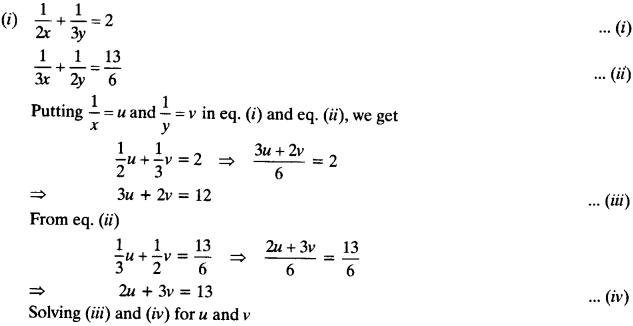By cross multiplication methodBy cross multiplication method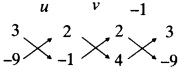By cross multiplication method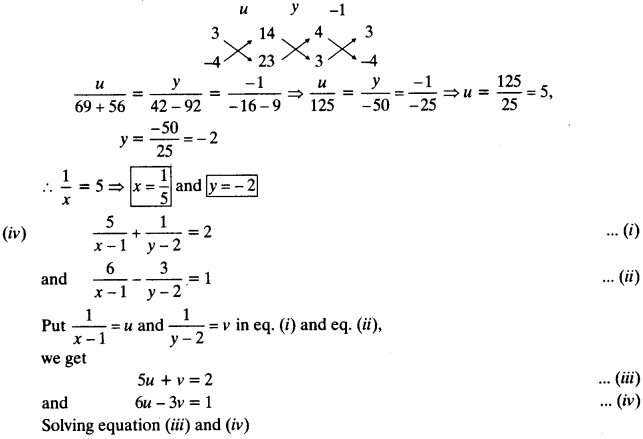By cross multiplication method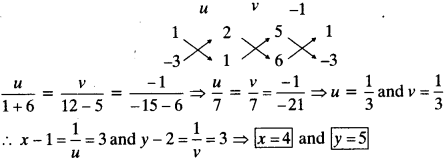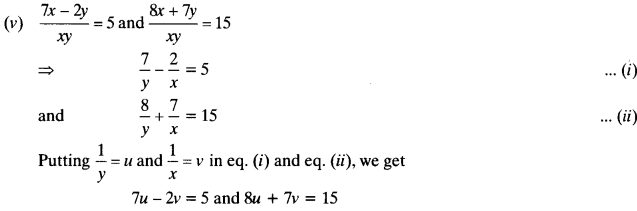solving for u and v by cross multiplication method:By cross multiplication method: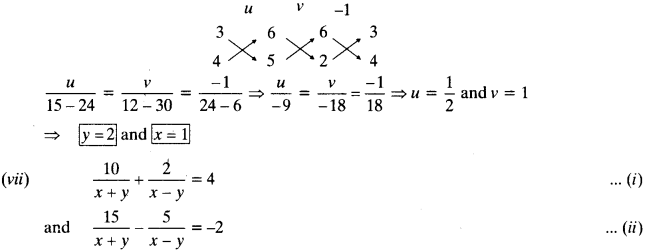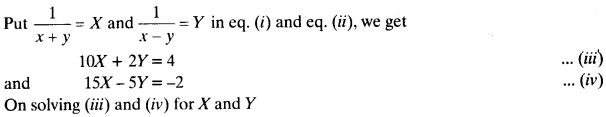By cross multiplication method: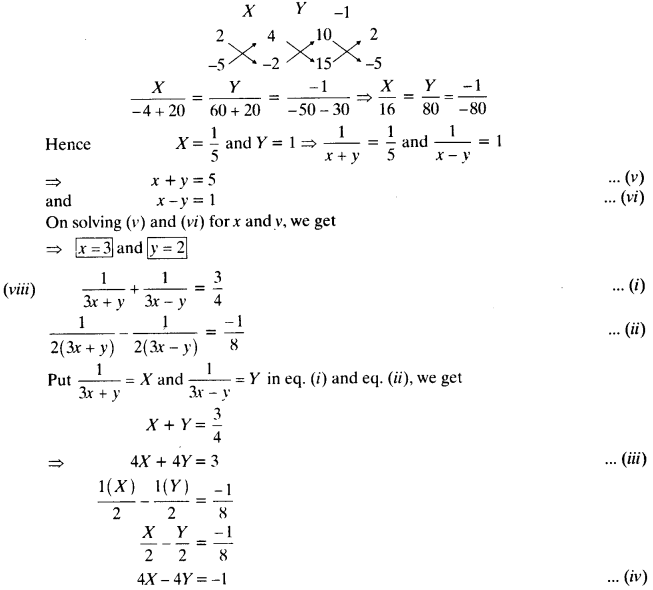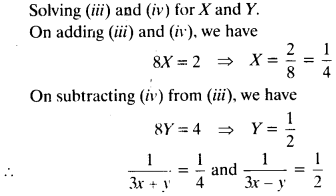NCERT Solutions For Class 10th Maths Chapter 3 Exercise 3.6 Question 2.
Formulate the following problems as a pair of equations, and hence find their solutions:
(i) Ritu can row’ downstream 20 km in 2 hours, and upstream 4 km in 2 hours. Find her speed of rowing in still water and the speed of the current.
(ii) 2 women and 5 men can together finish an embroidery work in 4 days, while 3 women and 6 men can finish it in 3 days. Find the time taken by 1 woman alone to finish the work, and also that taken by 1 man alone.
(iii) Roohi travels 300 km to her home partly by train and partly by bus. She takes 4 hours if she travels 60 km by train and the remaining by bus. If she travels 100 km by train and the remaining by bus, she takes 10 minutes longer. Find the speed of the train and the bus separately.
Solution:
(i) Let Ritu’s speed in still water = x km/h
Speed of current = y km/h
During downstream, speed = (x + y) km/h
During upstream, speed = (x -y) km/h
A.T.Q.
1st condition :
x + y = 20/2 ⇒ x + y = 10
2nd condition :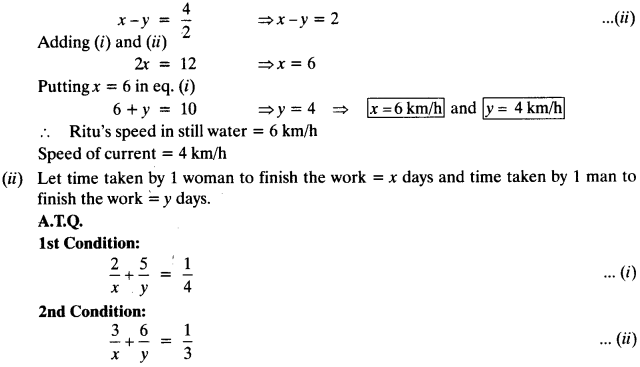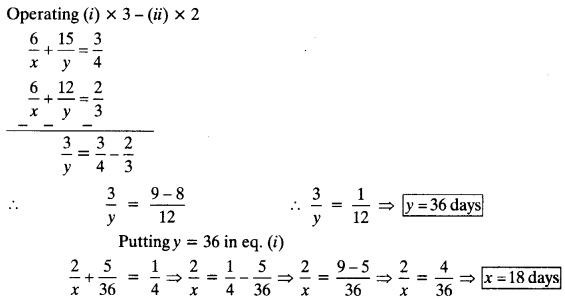Time taken by 1 woman to finish the work = 18 days.
Time taken by 1 man to finish the work = 36 days.

(iii) Let speed of train = x km/h and Speed of bus = y km/h
Total distance = 300 km
A.T.Q.
1st condition :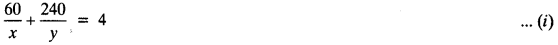2nd condition :We hope the NCERT Solutions for Class 10 Mathematics Chapter 3 Pair of Linear Equations in Two Variables Ex 3.6 help you. If you have any query regarding NCERT Solutions for Class 10 Mathematics Chapter 3 Pair of Linear Equations in Two Variables Ex 3.6, drop a comment below and we will get back to you at the earliest.